Students can Download Tamil Nadu 12th Chemistry Model Question Paper 4 English Medium Pdf, Tamil Nadu 12th Chemistry Model Question Papers helps you to revise the complete Tamilnadu State Board New Syllabus, helps students complete homework assignments and to score high marks in board exams.

## TN State Board 12th Chemistry Model Question Paper 4 English Medium

Time: 3 Hours
Maximum Marks: 70

Instructions:

1. The question paper comprises of four parts
2. You are to attempt all the parts An internal choice of questions is provided wherever applicable
3. All questions of Part I, II, III, and IV are to be attempted separately
4. Question numbers 1 to 15 in Part I are Multiple Choice Questions of one mark each. These are to be answered by choosing the most suitable answer from the given four alternatives and writing the option code and the corresponding answer
5. Question numbers 16 to 24 in Part II are two-mark questions. These are lo be answered in about one or two sentences
6. Question numbers 25 to 33 in Part III are three-marks questions. These are to be answered in about three to five short sentences
7. Question numbers 34 to 38 in Part IV are five-mark Questions These are to answered in detail. Draw diagrams wherever necessary

Part – 1

Answer all the questions. Choose the correct answer. [15 × 1 = 15]

Question 1.
Depressing agents used to separate ZnS from PbS is …………..
(a) NaCN
(b) NaCl
(c) NaNO3
(d) NaNO2
(a) NaCN

Question 2.
The basic structural unit of silicates is
(a)(SiO3)2-
(b) (SiO4)2-
(c) (SiO)
(d) (SiO4)4-
(d) (SiO4)4-Question 3.
………………… is a pungent smelling gas.
(a) Ammonia
(b) Nitric acid
(c) Fluorite
(d) Sodium chloride
(a) Ammonia

Question 4.
Consider the following statement.
(i) All the actinoids are non radioactive.
(ii) Neptunium and other heavier elements are produced by artificial transformation of naturally occurring elements by nuclear reactions.
(iii) Most.of the actinoids have long half lives.
Which of the above statements is/are not correct.
(a) i only
(b) i and ii
(c) ii and iii
(d) i and iii
(d) i and iii

Question 5.
How many geometrical isomers are possible for [Pt (Py) (NH3) (Br) (Cl)]?
(a) 3
(b) 4
(c) 0 3
(d) 15
(a) 3

Question 6.
Metal excess defect is possible in……………….
(a) AgCl
(b) AgBr
(c) KCl
(d) Fes
(c) KCl

Question 7.
The correct difference between first and second order reactions is that ……………………
(a) A first order reaction can be catalysed; a second order reaction cannot be catalysed.
(b) The half life of a first order reaction does not depend on [A0]; the half-life of a second order reaction does depend on [A0].
(c) The rate of a first order reaction does not depend on reactant concentrations; the rate of a second order reaction does depend on reactant concentrations.
(d) The rate of a first order reaction does depend on reactant concentrations; the rate of a second order reaction does not depend on reactant concentrations.
(b) The half life of a first order reaction does not depend on [A0]; the half-life of a second order reaction does depend on [A0].
Solution:
For a first order reaction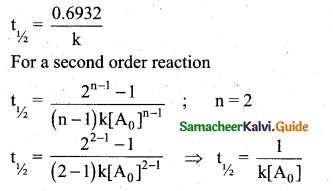Question 8.
Concentration of the Ag+ ions in a saturated solution of Ag2C2O4 is 2.24 × 10-4 mol L-1 solubility product of Ag2C2O4 is
(a) 2.42 × 10-8 mol3 L-3
(b) 2.66 × 10-12mol3 L-3
(c) 4.5 × 10-11mol3 L-3
(d) 5.619 × 10-12mol3 L-3
(d) 5.619 × 10-12mol3 L-3
Solution: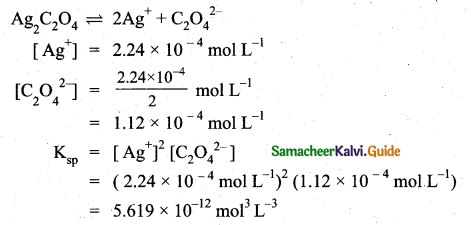Question 9.
Assertion(A): Copper Sulphate can be stored in a Zinc vessel.
Reason (R): Zinc is less reactive than Copper.
(a) Both A and R are correct
(b) Both A and R are wrong
(c) A is correct but R is wrong
(d) A is wrong but R is correct
(b) Both A and R are wrong

Question 10.
Fog is colloidal solution of……………..
(a) solid in gas
(b) gas in gas
(c) liquid in gas
(d) gas in liquid
(c) liquid in gas

dispersion medium-gas
dispersed phase-liquid

Question 11.
Match the following Column-I with Column-II using the code given below.
c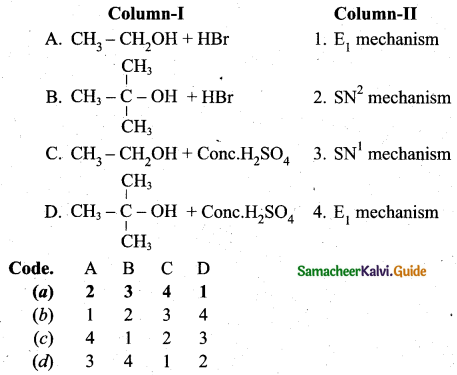(a) A – 2,B – 3, C – 4, D – 1

Question 12.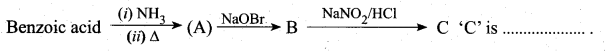(a) anilinium chloride
(b) O – nitro aniline
(c) benzene diazonium chloride
(d) m – nitro benzoic acid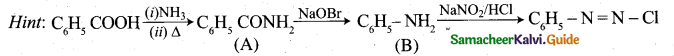(c) benzene diazonium chloride

Question 13.
Which one of the following is the IUPAC name of CH3 – CH2 – CH2 CN?
(a) Propiono nitrile
(b) Butane cyanide
(c) Isobutyro nitrile
(d) Butane nitrile
(d) Butane nitrile

Question 14.
The correct corresponding order of names of four aldoses with configuration given below Respectively is ……………
(a) L-Erythrose, L-Threose, L-Eiythrose, D-Threose
(b) D-Threose, D-Erythrose, L-Threose, L-Erythrose
(c) L-Erythrose, L-Threose, D-Erythrose, D-Threose
(d) D-Erythrose, D-Threose, L-Erythrose, L-Threose
(d) D-Erythrose, D-Threose, L-Erythrose, L-Threose

Question 15.
Tranquilisers are substances used for the treatment of ………………
(a) cancer
(b) AIDS.
(c) mental diseases
(d) blood infection
(c) mental diseases

Part – II

Answer any six questions. Question No. 22 is compulsory. [6 × 2 = 12]

Question 16.
What are leaching process?
This method is based on the solubility of the ore in a suitable solvent and the reactions in aqueous solution. In this method, the crushed ore is allowed to dissolve in a suitable solvent, the metal present in the ore is converted to its soluble salt or complex while the gangue remains insoluble. This process is also called chemical method.Question 17.
What happens when PCI5 is heated?
On heating phosphorous pentachloride, it decomposes into phosphorus trichloride and chlorine.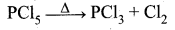Question 18.
Write the biologically importance of coordination compounds.
Biological important of coordination compounds:

(i) Ared blood corpuscles (RBC) is composed of heme group, which is Fe2+– Porphyrin complex, it plays an important role in carrying oxygen from lungs to tissues and carbon dioxide from tissues to lungs.

(ii) Chlorophyll, a green pigment present in green plants and algae, is a coordination complex containing Mg2+ as central metal ion surrounded by a modified Porphyrin ligand called corrin ring. It plays an important role in photosynthesis, by which plants converts CO2 and water into carbohydrates and oxygen.

(iii) Vitamin B12(cyanocobalamine) is the only vitamin consist of metal ion. it is a coordination complex in which the central metal ion is CO+ surrounded by Porphyrin like ligand.

(iv) Many enzymes are known to be metal complexes, they regulate biological processes. For example, Carboxypeptidase is a protease enzyme that hydrolytic enzyme important in digestion, contains a zinc ion coordinated to the protein.

Question 19.
Mention the factors affecting the reaction rate.
The rate of the reaction is affected by the following factors.

• Nature and state of the reactant .
• Concentration of the reactant
• Surface area of the reactant
• Temperature of the reaction
• Presence of a catalyst

Question 20.
What is meant by standard reduction potential? What is its application?

• The standard reduction potential (E°) is a measure of the oxidising tendency of the species.
• The greater the E° value means greater is the tendency shown by the species to accept electrons and undergo reduction.
• So higher the E° values, lesser is the tendency to undergo corrosion.

Question 21.
Give two important characteristics of physisorption.
Important characteristics of physisorption:

• It is reversible
• It has low heat of adsorption
• It has weak van der Waals forces of attraction with adsorbent.
• It increases with increase in pressure.
• It forms multimolecular layer.

Question 22.
Draw the major product formed when 1-ethoxyprop-l-ene is heated with one equivalent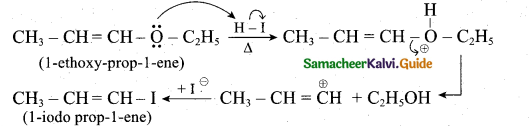This reaction follows SN1 mechanism because in this reaction the more stable carbocation is formed that is double bonded carbocation. Therefore, the given molecule reacts with HI to form ethanol and 1-iodo prop-1-ene.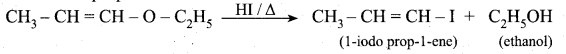Question 23.
Define Tautomerism. Give example. Why tertiary nitro alkanes do not exhibit tautomerism?
(i) Tautomerism is an isomerism in which the isomers change into one another with great ease of shifting of proton so that they exist together in equilibrium.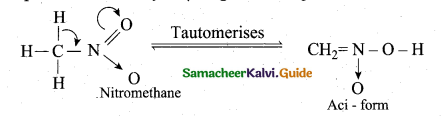(ii) Tertiary nitro alkanes do not exhibit tautomerism due to absence of a-H atom.

Question 24.
Why do soaps not work in hard water?
Soaps are sodium or potassium salts of long-chain falty acids. Hard water contains calcium and magnesium ions. When soaps are dissolved in hard water, these ions displace sodium or potassium from insoluble calcium or magnesium salts of fatty acids. These insoluble salts separate as scum.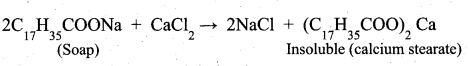This is the reason why soaps do not work in hard water.

Part – III

Answer any six questions. Question No. 31 is compulsory. [6 × 3 = 18]

Question 25.
Write the application of Iron (Fe).

• Iron is one of the most useful metals and its alloys are used everywhere including bridges, electricity pylons, bicycle chains, cutting tools and rifle barrels.
• Cast iron is used to make pipes, valves and pumps stoves etc.
• Magnets can be made of iron and its alloys and compounds.

An important alloy of iron is stainless steel, and it is very resistant to corrosion. It is used in architecture, bearings, cutlery, surgical instruments and jewellery. Nickel steel is used for making cables, automobiles and aeroplane parts. Chrome steels are used for manufacturing cutting tools and crushing machines.

Question 26.
Give a reason to support that sulphuric acid is a dehydrating agent.
Sulphuric acid is highly soluble in water and has strong affinity towards water and hence it can be used as a dehydrating agent. When dissolved in water it forms mono (H2SO4.H2O) and di (H2SO4.H2O) hydrates and the reaction is exothermic.
The dehydration property can also be illustrated by its reaction with organic compounds such as sugar, oxalic acid and formic acid.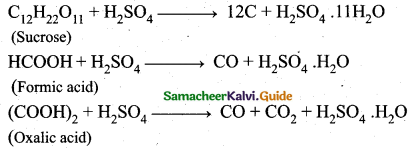Question 27.
Give evidence that [CO(NH3)gCl]SO4 and [CO(NH3)5SO4]Cl are ionisation isomers.
When they are dissolved in water, they will give different ions in the solution which can be
tested by adding AgNO3 solution and BaCl2 solution. When Cl ions are the counter ions, a white precipitate will be obtained with AgNO3 solution. If SO42- ions are the counter ions, a white precipitate will be obtained with BaCl2 solution.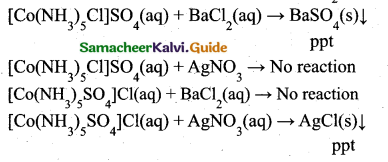Question 28.
For a reaction, X + Y → Product; quadrupling [x] , increases the rate by a factor of 8. Quadrupling both [x] and [y], increases the rate by a factor of 16. Find the order of the reaction with respect to x and y. what is the overall .order of the reaction?z = k [x]m [y]n …… (1)
8z = k [4x]m [y]n…… (2)
I 6z = k [4x]m [4y]n…… (3)
Dividing Eq (2) by Eq (1) we get,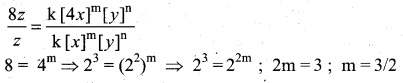1.5 order with respect to x.
Dividing Eq (3) by Eq (1) we get,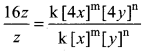16 = 4m . 4n
16 = 42.4n
16/16 = 4n
1 = 4n
∴ n = 0 [Zero order with respect toy]
Overall order of the reaction, ‘
k[x]m[y]n
k [x]1.5 [y]0
Order = (1.5 + 0) = 1.5

Question 29.
Identify the conjugate acid base pair for the following reaction in aqueous solution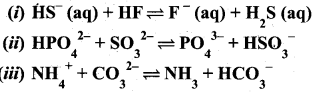Answer: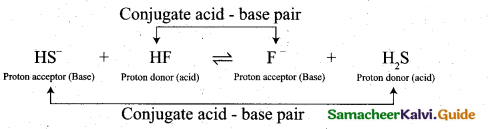• HF and F , HS and H2S are two conjugate acid – base pairs.
• F is the conjugate base of the acid HF (or) HF is the conjugate acid of F .
• H2S is the conjugate acid of HS (or) HS is the conjugate base of H2S.

(ii)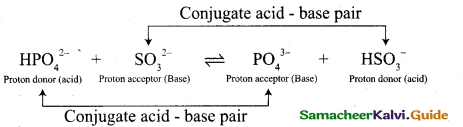• HPO42- and PO43- , SO3-2 and HSO3 are two conjugate acid – base pairs.
• PO43- is the conjugate base of the acid HPO42- (or)
HPO42- is the conjugate acid of PO43-.
• HSO3 is the conjugate acid of SO3-2 (or) SO3-2 is the conjugate base of HSO3

(iii)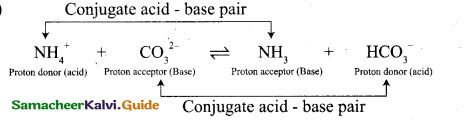• NH4+ and NH3, CO32- and HCO3 are two conjugate acid – base pairs.
• HCO3 is the conjugate of acid CO32- (or) CO32- is the conjugate bases of HCO3
• NH3 is the conjugate base of NH4+ (or) NH4+ is the conjugate acid of NH3.

Question 30.
In the following fields, how adsorption is applied?
(i) Medicine (ii) Metallurgy (iii) Mordant & Dyes (iv) indicators
(i) Medicine: – Drugs cure diseases by adsorption on body tissues.

(ii) Metallurgy:- Sulphide ores are concentrated by a process called froth floation in which lighter ore particles are adsorbed by pine oil.

(iii) Mordants and Dyes:- Most of the dyes are adsorbed on the surface of the fabric. Mordants are the substances used for fixing dyes onto the fabric.

(iv) In the precipitation titrations, the end point is indicated by an external indicator which changes its colour after getting absorbed on precipitate. It is used to indicate the end point of filtration.Question 31.
Identify A, B, C, and D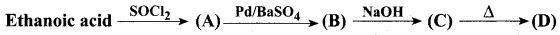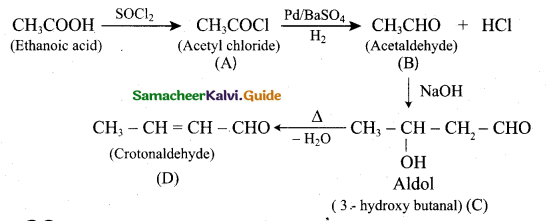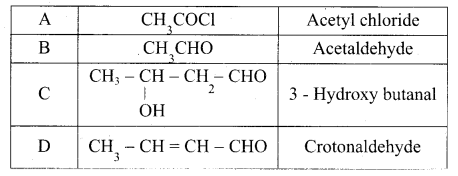Question 32.
Draw the structural formula and write the IUPAC name of
(i) N, N-dimethyl aniline (ii) Benzyl amine (iii) N-methyl benzylamine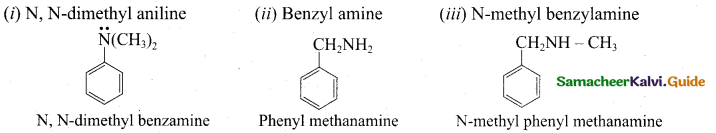Question 33.
Differentiate soap and detergents?
Soap :

1. Soaps are sodium or potassium salt of long chain fatty acid.
2. Soaps are made from animal (or) plant fats and oils.
3. Soaps have lesser cleansing action.
4. Soaps are bio degradable.
5. Soaps are less effective in hard water.
6. They have a tendency to form a scum in hard water.
7. Example: Sodium palmitate.

Detergent :

1. Detergent is sodium salt of alkyl hydrogen sulphate or alkyl benzene sulphonic acid.
2. Detergents are made from petrochemicals.
3. Detergents have more cleansing action.
4. Detergents are non-bio degradable.
5. Detergents are more effective even in hard water.
6. They do not form scum with hard water.
7. Example: Sodium lauryl sulphate.

Part – IV

Answer all the questions. [5 × 5 = 25]

Question 34.
(a) (i) Explain the role of carbon monoxide in the purification of nickel? (2)
(ii) Describe the structure of diborane. (3)
[OR]
(b) (i) What type of hybridisation occur in 1. BrF5. 2. BrF3 (2)
(ii) Most of the transition metals act as catalyst. Justify this statement. (3)
(a) (i) During the purification of Nickel by Mond’s process, carbon monoxide (CO) is used to convert impure nickel to nickel carbonyl.
Nickel carbonyl is an unstable compound. Heating to higher temperature decomposes it to give pure nickel.

(ii) In diborane two BH2 units are linked by two bridged hydrogens. Therefore, it has eight B-H bonds. However, diborane has only 12 valance electrons and are not sufficient to
form normal covalent bonds. The four terminal B-H bonds are normal covalent bonds (two centre – two electron bond or 2c-2e bond). The remaining four electrons have to used for the bridged bonds.
1. e. two three centred B-H-B bonds utilise two electrons each. Hence, these bonds are three centre- two electron bonds. The bridging hydrogen atoms are in a plane as shown in the figure.

In dibome, the boron is sp3 hybridised. Three of the four sp3 hybridised orbitals contains single electron and the fourth orbital is empty. Two of the half filled hybridised orbitals of each boron overlap with the two hydrogens to form four terminal 2c-2e bonds, leaving one empty and one half filled hybridised orbitals on each boron. The Three centre – two electron bonds, B-H-B bond formation involves overlapping the half filled hybridised orbital of one boron, the empty hybridised orbital of the other boron and the half filled Is orbital of hydrogen.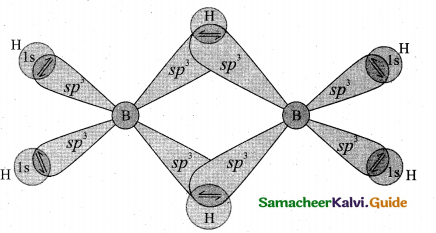[OR]

(b) (i) 1. BrF5. is a AX5 type. Therefore is has sp3 d2 hybridisation. Hence, BrF5 molecule has square pyramidal shape.
2. BrF3 is a AX3 type. Therefore it has sp3 d hybridisation. Hence, BrF3 molecule has T-shape.

(ii) Many industrial processes use transition metals or their compounds as catalysts. Transition metal has energetically available d orbitals that can accept electrons from reactant molecule or metal can form bond with reactant molecule using its ‘d’ electrons.

For example, in the catalytic hydrogenation of an alkene, the alkene bonds to an active site by using its n electrons with an empty d orbital of the catalyst.

Question 35.
(a) (i) Why tetrahedral complexes do not exhibit geometrical isomerism. (2)
(ii) Explain about the importance and application of coordination complexes. (3)
[OR]
(b) Explain AAAA and ABABA and ABCABC type of three dimensional packing with the help of neat diagram.
(a) (i) In tetrahedral geometry

• AH the four ligands are adjacent or equidistant to one another.
• The relative positions of donor atoms of ligands attached to the central metal atom are same with respect to each other.
• It has plane of symmetry

Therefore, tetrahedral complexes do not exhibit geometrical isomerism.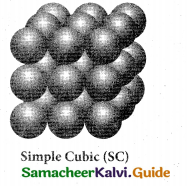(ii) 1. Phthalo blue – a bright blue pigment is a complex of copper (II) ion and it is used in printing ink and packaging industry.

2. Purification of Nickel by Mond’s process involves formation of [Ni (CO)4 ] which yields 99.5% pure on decomposition.

3 . EDTA is used as a chelating ligand for the separation of lanthanides, in softening of hard water and also in removing lead poisoning.

4. Coordination complexes are used in the extraction of silver and gold from their ores
by forming soluble cyano complex. These cyano complexes are reduced by zinc to
yield metals. This process is called Mac – Arthur Forrest cyanide process.

5. Some metal ions are estimated more accurately by complex fonnation. For eg., Ni present in Nickel chloride solution is estimated accurately forming an insoluble, complex called [Ni (DMG)2].

6. Many of the complexes are used as catalyst in organic and inorganic reactions. For e.g.,
(i) Wilkinson’s Catalyst – [(PPh3)3, Rh Cl] is used for hydrogenation of alkenes.
(ii) Ziegler – Natta Catalyst [TiCl4 + Al (C2H5) ]3 is used in the polymerisation of ethene.

7. In photography, when the developed film is washed with sodium thio sulphate solution (hypo), the negative film gets fixed. Undecomposed AgBr forms a soluble complex called sodium dithio sulphate argentate (I) which can be removed easily by washing the film with water.

AgBr + 2 Na2 S2O3 → Na3 [Ag (S2O3)2] + 2NaBr

[OR]

(b) (i) AAAA type of three dimensional packing:
This type of three dimensional packing arrangements can be obtained by repeating the AAAA type two dimensional arrangements in three dimensions, i.e., spheres in one layer sitting directly on the top of those in the previous layer so that all layers are identical. All spheres of different layers of crystal are perfectly aligned horizontally and also vertically, so that any unit cell of such arrangement as simple cubic structure as shown in fig.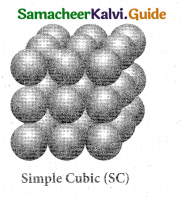In simple cubic packing, each sphere is in contact with 6 neighbouring spheres – Four in its own layer, one above and one below and hence the coordination number of the sphere in simple cubic arrangement is 6.

(ii) ABABA type of three dimensional packing:
In this arrangement, the spheres in the first layer (A type) are slightly separated and the second layer is formed by arranging the spheres in the depressions between the spheres in layer A as shown in figure. The third layer is a repeat of the first. This pattern ABABAB is repeated throughout the crystal. In this arrangement, each sphere has a coordination number of 8, four neighbors in the layer above and four in the layer below.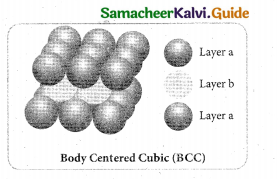(iii) ABCABC type of three dimensional packing:
In this arrangement (FCC) second layer spheres are arranged at the dips of first layer. Third layer spheres are arranged in a manner such that it cover the octahedral void. Then no longer third layer is similar to first or second layer. Third layer gives different arrangement. Fourth Layer spheres are similar to first layer. If the first, second and third layer are represented as A,B,C then this type of packing gives the arrangement of layers as ABCABC… (Le.,), the first three layers do not resemble first, second and third layers respectively and the sequence is repeated with the addition of more layers.

In this arrangement atoms occupy 74% of the available space and thus has 26% vacant space. The coordination number is 12.

Voids: The empty spaces between the three dimensional layers are known as voids. There are
two types of common voids possible. They are tetrahedral and octahedral voids.

Tetrahedral void:
A void formed by three spheres of a layer in contact with each other and also with a sphere on the top or bottom r Layer a layer is a hole between four spheres. The spheres are arranged at the vertices Layerc of a regular tetrahedron such a hole or void is called tetrahedral void.

Octahedral void:
A hole or void formed by three spheres of a hexagonal layer and another three spheres of the adjacent layer is a hole between six spheres. The spheres are arranged at the vertices of a regular octahedron. Such a hole or void is abc arrangement – ccp structure called octahedral void.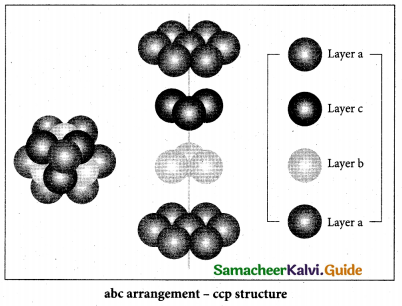Question 36.
(a) (i) The rate constant for a first order reaction is 1.54 × 10-3s-1 (2)
Calculate its half life time.
(ii) Explain about protective action of colloid. (3)
[OR]
(b) (i) What is buffer solution? Give an example for an acidic buffer and a basic buffer. (2)
(ii The value of kspof two sparingly soluble salts Ni(OH)2 and AgCN are 2.0 × 10-15 and 6 × 10-17 respectively. Which salt is more soluble? Explain. (3)
(a) (i) We know that, t1/2 = 0.693/ k
t1/2= O.693/1.54 ×10-3s -1 = 450s

(ii) 1. Lyophobic sols are precipitated readily even with small amount of electrolytes. But they are stabilised by the addition of small amount of lyophillic colloid.

2. A smaLl amount of gelatine sol is added to gold sol to protect gold sol.

3. Gold number is a measure of protecting power of a colloid. Gold number is defined as the number of milligrams of hydrophillic colloid that will just prevent the precipitation of 10 ml of gold sol on the addition of ImI of 10% NaCI solution. Smaller the gold number, greater the protective power.

[OR]

(b) (i) 1. Buffer is a solution which consists of a mixture of weak acid and its conjugate base (or) a weak base and its conjugate acid.

2. This buffer solution resists drastic changes in its pH upon addition of a small quantities of acids (or) bases and this ability is called buffer action.

3. Acidic buffer solution : Solution containing acetic acid and sodium acetate.
Basic buffer solution : Solution containing NH4O and NH4Cl.

(ii)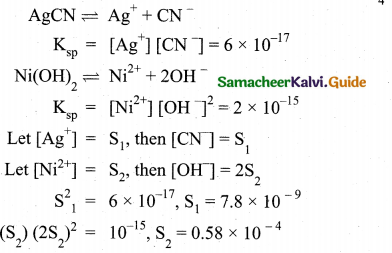Ni(OH)2 is more soluble than AgCN.

Question 37.
(a) Describe about lead storage battery construction and its uses. (5)
[OR]
(b) (i) What happens when m-cresol is treated with acidic solution of sodium dichromate? (3)
(ii) Formic acid is more stronger than acetic acid. Justify this statement. (2)
(a) Lead storage battery :
1. Anode : Spongy lead
Cathode : Lead plate bearing PbO2
Electrolyte : 38% by mass of H2SO4 with density 1.2 g/ml

2. Oxidation occurs at the anode

Pb(s) → Pb2+ (aq) + 2e …………(1)

The Pb2+ ions combine with SO42- to form PbSO4 precipitate

Pb2+(aq) + SO42- → PbS4(s) …………….(2)

3. Reduction occurs at the cathode

PbO2(s) + 4H+(aq) + 2e – → Pb2+(aq) + 2H2O ………….(3)

The Pb2+ ions also combines with SO42- ions to form sulphuric acid to form PbSO4 Precipitate

Pb2+(aq) + SO42-(aq) → PbSO4 …………..(4)

4. The overall reaction is,
(1) + (2) + (3) + (4)
pb(s) + PbO2(s) + 4H+ + 2SO42-(aq) → 2PbSO4(s) + 2H2O(l)

5. The emf of a single cell is about 2V. Usually six such cells are combined in series to produce 12 volts.

6. The emf of the cell depends on the concentration of H2SO4. As the cell reaction uses SO42- ions, the concentration H2SO4 decreases. When the cell potential falls to about 1,8V, the cell has to be recharged.

7. Recharge of the cell: During recharge process, the role of anode and cathode is reversed and H2SO4 is regenerated.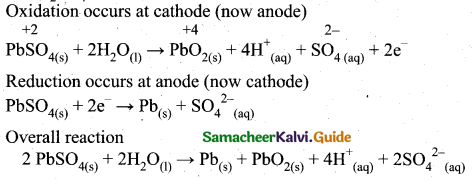The above reaction is exactly the reverse of redox reaction which takes place while discharging.

8. Uses: Lead storage battery is used in automobiles, trains, inverters.

[OR]

(b) (i) When m-cresol is treated with acidic solution of sodium dichromate it gives 4-hydroxy benzoic acid.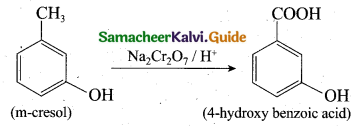(ii) The electron releasing groups (+1 groups) increase the relative charge on the carboxylate ion and destabilise it and hence the loss of proton becomes difficult.
+I groups are CH3, – C2H5, – C3H7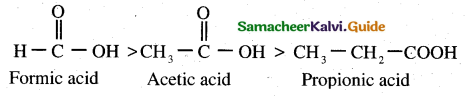Question 38.
(i) An aromatic compound ‘A’ on treatment with aqueous ammonia and heating
forms compound ‘B’ which on heating with Br2 and KOH forms a compound ‘C’
of molecular formula C6H7N. Write the structures and IUPAC names of compound
A,BandC. (3)
(ii) Lactose act as reducing sugar. Justify this statement. (2)
[OR]
(b) (i) Explain the mechanism of enzyme action? (3)
(ii) Which chemical is responsible for the antiseptic properties of dettol? (2)
(a) (i) Step-1: To find out the structure of compounds‘B’and‘C’.
1. Since compound ‘C’ with molecular formula C6H7N is formed from compound ‘B’ on treatment with Br2 + KOH (i.e, ., Hoffmann bromamide reaction). Therefore, compound ‘B’ must be an amide and ‘C’ must be an amine. The only amine having the molecular formula C6H7N is C6H5NH2 (i.e., aniline or benzenamine).

2. Since ‘C’ is aniline, therefore, the amide from which it is formed must be benzamide (C6H5CONH2). Thus, compound ‘B’ is benzamide:
The chemical equation showing the conversion of ‘B’ to ‘C’ is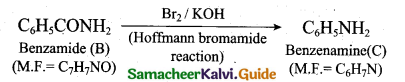Step-2: To find out the structure of compound ‘A’. Since compound ‘B’ is formed from compound ‘A’ by treatment with aqueous ammonia and heating. Therefore, compound ‘A’ must be benzoic acid or benzenecarboxylic acid.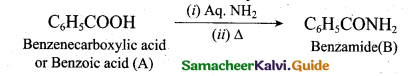(ii) Lactose is a disaccharide and contains one galactose unit and one glucose unit.
In lactose, the β-D galactose and β-D glucose are linked by β-1, 4 – glycosidic bond.
The aldehyde carbon is not involved in the glycosidic bond hence it retains its reducing property and is called a reducing sugar.

[OR]

(b) (i) Enzymes are bio catalysts that catalyse a specific bio chemical reaction. They generally activate the reaction by reducing the activation energy by stabilising the transition state.

In a typical reaction, enzyme E binds with the substrate molecule leversity to produce an enzyme substrate complex. During this stage the substrate is converted into product and the enzyme becomes free and is ready to bind to another substrate molecule.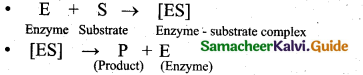(ii) (a) Chloroxylenol and (b) Terpineol are the chemicals responsible for the antiseptic properties of dettol. But among these two, chloroxylenol plays more important role. Chloroxylenol is an antiseptic and disinfectant which is used for skin disinfection and cleaning surgical instruments.